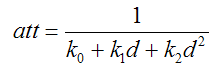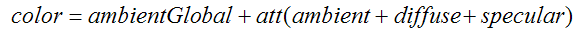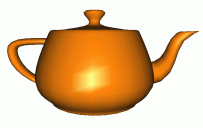Prev: Dir. Light per Pixel Next: Spot Light Per Pixel

This tutorial is based on the directional lights tutorial as most of the code comes from there. The tutorial is based on the difference between a directional light and a point light. A directional light is assumed to be infinitely far away, so that the light rays are parallel when they reach the object. In contrast, a point light has a position, and sends rays in all directions. Furthermore, in a point light, the intensity decays with the distance to the vertex.

From an OpenGL application point of view there are two differences between the two:

• the w component of the light position field: in a directional light it is zero to indicate that the position is in fact a direction (or vector), where as in a point light the w component of the light position field is 1.
• The attenuation is specified based on three coefficients: a constant term, a linear term, and a quadratic term

From a computational point of view these differences must be taken care of. For a directional light, the direction of the light rays is constant for every vertex, whereas for a point light it is the vector from the vertex to the lights position. Hence, all that needs to change in the vertex shader is the computation of the lights direction.

The attenuation is computed based on the following formula in OpenGL:where k0 is the constant attenuation, k1 is the linear attenuation, k2 is the quadratic attenuation and d is the distance from the light’s position to the vertex.

Note that the attenuation does not vary linearly with distance, hence we can’t compute the attenuation per vertex and use the interpolated value in the fragment shader. We can however compute the distance in the vertex shader and use the interpolated distance in the fragment shader to compute the attenuation.

The equation for the color using a point light is:As shown in the above equation, the ambient term must be spitted in two: one global ambient term using the lighting model ambient setting and a light specific ambient term. The vertex shader must separate the computation of the ambient term accordingly. The new vertex shader is:

```varying vec4 diffuse,ambientGlobal,ambient, ecPos;
varying vec3 normal,halfVector;

void main()
{
vec3 aux;

/* first transform the normal into eye space and normalize the result */
normal = normalize(gl_NormalMatrix * gl_Normal);

/* compute the vertex position  in camera space. */
ecPos = gl_ModelViewMatrix * gl_Vertex;

/* Normalize the halfVector to pass it to the fragment shader */
halfVector = gl_LightSource.halfVector.xyz;

/* Compute the diffuse, ambient and globalAmbient terms */
diffuse = gl_FrontMaterial.diffuse * gl_LightSource.diffuse;
ambient = gl_FrontMaterial.ambient * gl_LightSource.ambient;
ambientGlobal = gl_LightModel.ambient * gl_FrontMaterial.ambient;

gl_Position = ftransform();
}
```

The fragment shader needs to compute the attenuation.

```varying vec4 diffuse,ambientGlobal, ambient, ecPos;
varying vec3 normal,halfVector;

void main()
{
vec3 n,halfV,viewV,lightDir;
float NdotL,NdotHV;
vec4 color = ambientGlobal;
float att, dist;

/* a fragment shader can't write a verying variable, hence we need
a new variable to store the normalized interpolated normal */
n = normalize(normal);

// Compute the ligt direction
lightDir = vec3(gl_LightSource.position-ecPos);

/* compute the distance to the light source to a varying variable*/
dist = length(lightDir);

/* compute the dot product between normal and ldir */
NdotL = max(dot(n,normalize(lightDir)),0.0);

if (NdotL > 0.0) {

att = 1.0 / (gl_LightSource.constantAttenuation +
gl_LightSource.linearAttenuation * dist +
color += att * (diffuse * NdotL + ambient);

halfV = normalize(halfVector);
NdotHV = max(dot(n,halfV),0.0);
color += att * gl_FrontMaterial.specular * gl_LightSource.specular * pow(NdotHV,gl_FrontMaterial.shininess);
}

gl_FragColor = color;
}
```

The following images show the difference between a point light as computed by the fixed functionality, i.e. per vertex, and using the shader in this tutorial, i.e. per pixel.Fixed Functionality Per Pixel

 Prev: Dir. Light per Pixel Next: Spot Light Per Pixel

### 15 Responses to “Point Light Per Pixel”

1.Hello,

Can you post a sample C/C++ code that is using those shaders ? I haven’t to much experience with OpenGL and I don’t know what parameters should I send to the shaders.

Thank you,
Cosmin

2.I’m sorry, lightDir is defined here but it is not in the next chapter. Also viewV is defined but never used.

3.lightDir is not defined and dist is wrong too.

“We can however compute the distance in the vertex shader and use the interpolated distance in the fragment shader to compute the attenuation.”
Isn’t that going to decrease quality because distance is not linear function?

4.Thank you for what you’re offering!
dist is declared as varying and you’re writing to it in the fragment shader.

5.I get this error in the Fragment Shader.

ERROR: 0:20: Left-hand-side of assignment must not be read-only

What’s causing this problem?

•In fragment shader is variable “dist” as varying and varying variables are read-only in fragment shader. But I dont know why is it there, beacuse it is not used in vertex shader so u can delete that lines from both sahders.

Delete from fragment and vertex:
varying float dist;

float dist = length(lightDir);

•Fixed. Thanks for reporting the bug.

6.You’ve added the ambient inside the NdotL > 0.0 condition. Doesn’t this mean material ambience will never get used on shaded areas, only global ambience?

•Hi Mike,

Yes, but I’m using a global ambient term to compensate for it. But you’re right, you could also skip this global ambient term and put the ambient component outside the if statement.

Antonio

7.halfVector = gl_LightSource.halfVector.xyz;
is this line in vertext shader right?
for a point light, since halfvector = V – L, why is halfvector constant from pixel to pixel?

•The halfVector is therefore not constant per vertex, although the code may lead us to that conclusion. The halfVector is a derived field, and it is computed, by OpenGL, per vertex.

8.can u post tutorials for Shadow mapping ? it ll be very useful , since past 1 week i’v been searching for good tutorial for shadow mapping .

9.hi
i keep getting some black faces rendered in the geometry:
http://kineme.net/forum/General/GLSLproblemwithhardware#comment-23081

thanks for any help

10.11.